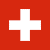Select Page

#Nernst equation (%O2 calculation)

## Calculation of oxygen concentration from CarboProbe sensor signal

Please enter the operating temperature (500 – 1700 deg C) and the measured mV (0 – 1200 mV) signal. The corresponding oxygen concentration is calculated. If it is very low, it is displayed in scientific notation.

The Nernst equation is E = (RT/nF)ln(pO2/pO2‘)

Where:
E = sensor electromotive force, (mV)
R = gas constant
T = temperature, (Kelvin)
n = number of charges per reactant species
F = Faraday constant
p = partial pressure (mole fraction)

This equation describes the electromotive force (emf) developed when there are different concentrations of a reactant on each side of an electrolyte.

If oxygen is to be measured, using a zirconia ceramic electrolyte and atmospheric air, with pO2 = 0.209, as a reference, this equation simplifies to:

pO2 = 0.209 exp(-46.421 E/T)

The equation may be re-arranged, to allow calculation of the emf from a known reference concentration and a measured oxygen concentration:

E = 0.0496 T log(pO2/0.209 )

ECONOX supplies a range of industrial, scientific and pottery probes for the measurement of oxygen at high temperature